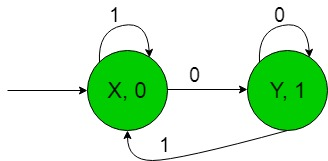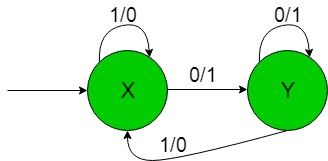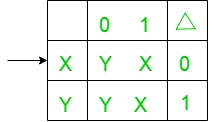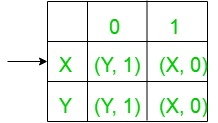GeeksforGeeks App
Open AppBrowser
Continue

# Finite Automata with Output (Set 11)

Prerequisite: Mealy and Moore Machines, Difference between Mealy machine and Moore machine
In this article, we will see some designing of Finite Automata with Output i.e, Moore and Mealy machines.

Problem: Construction of the machines that take the binary number {0, 1} as input and produce 1’s complement of that number as output.
Here the assumption is that the input string is read from LSB (Least Significant Bit) and end carry is discarded.
That is here we have,
Ε = {0, 1} and
Δ = {0, 1}
where Ε and Δ are the input and output alphabet respectively.

The required Moore machine is constructed below:Explanation:
In the above diagram, the initial state ‘X’ on getting ‘1’ as the input it remains in the state of itself and prints ‘0’ as the output and on getting ‘0’ as the input it transmits to a state ‘Y’ and prints ‘1’ as the output so on for the remaining states.

For example, when the input string is ‘101’ then above Moore machine produce output as ‘010’ which is nothing but 1’s complement of the given input i.e, 1 gets converted into 0 and vice versa.
Thus finally above Moore machine can easily produce 1’s complement of the given input binary string.

The required Mealy machine is constructed below:-Explanation:
In the above diagram, the initial state ‘X’ on getting ‘1’ as the input it remains in the state of itself and prints ‘0’ as the output and on getting ‘0’ as the input it transmits to a state ‘Y’ and prints ‘1’ as the output so on for the remaining states.

For example, when the input string is ‘101’ then above Moore machine produce output as ‘010’ which is nothing but 1’s complement of the given input i.e, 1 gets converted into 0 and vice versa.
Thus finally above Mealy machine can easily produce 1’s complement of the given input binary string.

Conversion from Moore machine to Mealy machine:
State Transition Diagram of a Moore machine:Above Moore machine takes the binary number {0, 1} as input and produce 1’s complement of that number as output.
Here the assumption is that the input string is read from LSB (Least Significant Bit) and end carry is discarded.

Now we need to transform the above transition diagram of Moore machine to equivalent Mealy machine transition diagram. Steps for the required conversion are given below:

• Step-1: Formation of State Transition Table of the above Moore machine-• In the above transition table, States ‘X’ and ‘Y’ are kept in the first column which on getting ‘0’ as the input it transits to ‘Y’ and ‘Y’ states respectively, kept in the second column and on getting ‘1’ as the input it transits to ‘X’ and ‘X’ states respectively, kept in the third column and In the fourth column under Δ, there are corresponding outputs of the first column states. In the table, An arrow (→) indicates the initial state.

• Step-2: Formation of Transition Table for Mealy machine from above Transition Table of Moore machine-
Below transition table is going to be formed with the help of the above table and its entries just by using the corresponding output of the states of the first column and placing them in the second and third column accordingly.• In the above table, the states in the first column like ‘X’ on getting ‘0’ as the input it goes to a state ‘Y’ and gives ‘1’ as the output and on getting ‘1’ as the input it goes to the state ‘X’ and gives ‘0’ as the output and so on for the remaining states in the first column. In the table, An arrow (→) indicates the initial state.

• Step-3: Then finally we can form the state transition diagram of Mealy machine with help of it’s above transition table.
The required diagram is shown below-• Above Mealy machine takes the binary number {0, 1} as input and produce 1’s complement of that number as output.
Here the assumption is that the input string is read from LSB (Least Significant Bit) and end carry is discarded.

Note: While converting from Moore to Mealy machine the number of states remains same for both Moore and Mealy machine but in case of Mealy to Moore conversion it does not give the same number of states.

My Personal Notes arrow_drop_up